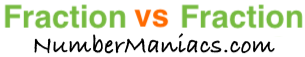1/3 vs 3/4People compare 1/3 vs 3/4 drywall, 1/3 vs 3/4 pipe, 1/3 vs 3/4 cup, 1/3 vs 3/4 ton, 1/3 vs 3/4 drill bit, 1/3 vs 3/4 poker, 1/3 vs 3/4 sump pump, 1/3 vs 3/4 garden hose, and many other items.

Is 1/3 greater than 3/4? Is 1/3 less than 3/4? What is the difference between 1/3 and 3/4? These and other questions are answered below so you can get a better perspective of 1/3 vs 3/4.

1/3 vs 3/4 Fractional Comparisons
1/3 is less than 3/4
The difference between 1/3 and 3/4 is 5/12
1/3 is 5/12 less than 3/4

1/3 vs 3/4 Decimal Comparisons
1/3 vs 3/4 as a decimal is 0.333 vs 0.75
The difference between 1/3 and 3/4 is 0.417
1/3 is 0.417 less than 3/4

1/3 vs 3/4 Percent Comparisons
1/3 vs 3/4 as a percent is 33.3% vs 75%
1/3 is 55.556 percent less than 3/4

Fraction vs Fraction
Submit another set of fractions that we can compare for you!

/
vs
/

The math behind the numbers
Calculations on this page are rounded to the nearest thousandth if necessary. Below is the math we used to analyze 1/3 vs 3/4:

To compare the size of 1/3 vs 3/4, we simply subtracted 3/4 from 1/3:

1/3 - 3/4 = |5/12|

To find 1/3 vs 3/4 as a decimal, we divided the numerator by the denominator for each fraction.

1 ÷ 3 = 0.333
3 ÷ 4 = 0.75

To find 1/3 vs 3/4 as a percent, we divided the numerator by the denominator and then multiplied the quotient by 100 for each fraction.

(1 ÷ 3) x 100 = 33.3%
(3 ÷ 4) x 100 = 75%

We found that 1/3 is 55.556 percent less than 3/4 by dividing the difference between the two fractions by the second fraction and then multiplied it by 100:

((5/12)/(3/4))×100 = 55.556%

That's it folks. We hope this page gave you a better understanding of 1/3 vs 3/4 with our explanations and conclusions.

1/3 vs 3/5
Here are the next two fractions we have compared and analyzed.